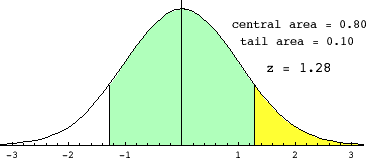# Range of the middle 75% of the data

## Homework Equations

TO find the kth percentile
=nk/100 (where k=kth percentile and n is the number of observations
Need n?

Given:
n(50)/100 = 9.3
n=18.6

Can n not be an integer?

Then I planned on plugging the n into the formula to solve
for the 75th percentile
n(75)/100 = range of the middle 75% of the data

Help

## The Attempt at a Solution

Last edited:

Redbelly98
Staff Emeritus
Homework Helper
Welcome to Physics Forums.

You'll need to calculate a z-statistic and use a table for the Standard Normal curve (which should be in your statistics textbook.)

A few things you should calculate or think about first:

1. What is the standard deviation for the dosage?
2. What percentage lies above the middle 75%? What percentage lies below the middle 75%?

Thank you so much. I think I understand

I want the x value that cuts off the upper 75% of the curve
P(X>x) = 0.75

So I went to the z-table, and got a z value of 0.67 (using 0.251 inside the table, or should i use 0.248)

0.67 = x-9.1/2.14
x= .67(2.14) + 9.1
x= 10.54

therefore, the range of the middle 75% of the data is at most 10.54

Redbelly98
Staff Emeritus
Homework Helper
Thank you so much. I think I understand

I want the x value that cuts off the upper 75% of the curve
P(X>x) = 0.75

So I went to the z-table, and got a z value of 0.67 (using 0.251 inside the table, or should i use 0.248)
Not quite. Take a look at this figure:If the middle (green part) of the area were 75%, what would be the areas of the white and yellow parts? And what would be the areas of the white+green combined?

0.67 = x-9.1/2.14
x= .67(2.14) + 9.1
x= 10.54

therefore, the range of the middle 75% of the data is at most 10.54
This part would be correct once you get the correct z-value to use.

Wait I think I have it now

There will be 12.5% between the mean and each Z-score,so we look for .125. The closest Z is .32, so the middle 75% is between Z = 0.32 and Z = -32.

Therefore, 9.1 - (0.32)(2.14) to 9.1 + (.32)(2.14) = 8.41 through 9.78.

Redbelly98
Staff Emeritus
Homework Helper
Wait I think I have it now

There will be 12.5% between the mean and each Z-score,...
Uh, no. There's 12.5% between each z-score and infinity.

Right, There will be 12.5% between infinity and each Z-score. There is 37.5% between each z-score and the mean

Don't i still look look for .125 making the middle 75% between Z = 1.15 and Z = -1.15. (from z-table)

Therefore, 9.1 - (1.15)(2.14) to 9.1 + (1.15)(2.14) = 6.61 through 11.6 making the range of the middle 75% of the data at most 4.99

Last edited:
Redbelly98
Staff Emeritus